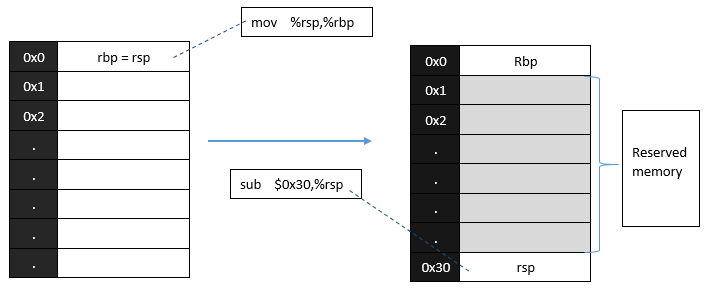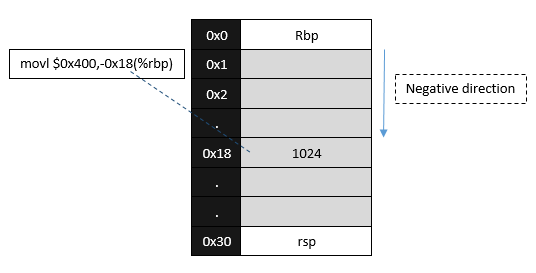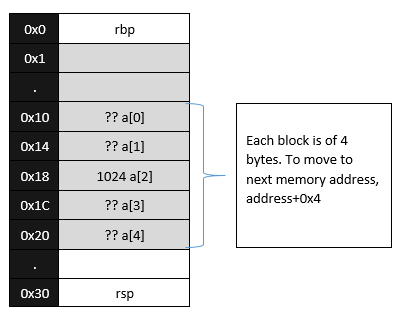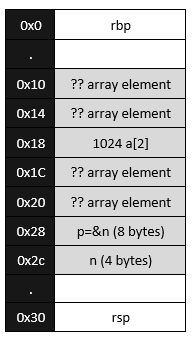# C to Assembly Language: First look

It’s actually really interesting and helping when you have total grasp over how things really work deep down in a computer. In order to really understand C language, you must also have in depth knowledge about assembly that is produced by the compiler.

As a first look, lets pick up a c program and try to set a goal for achieving something using knowledge of assembly language and memory allocation. All the files used in this tutorial can be found here.

`#include <stdio.h>int main(void) {     int n;   int a;   int *p;   a = 1024;   p = &n;   /*    * write your line of code here...    * Remember:   * - you are not allowed to use a    * - you are not allowed to modify p    * - only one statement    * - you are not allowed to code anything else    */       /* ...so that this prints 98\n */      printf("a = %d\n", a);      return (0);}`
`OUTPUT:a = 1024`

So we are allowed to put only one line in this code without using the variable a or changing the value of the pointer p. Our first initial check should be to check the size of these declared variables. So lets just check them in our c program by adding these lines in the main function:

`printf("size of n = %d\n", sizeof(n));printf("size of a = %d\n", sizeof(a));printf("size of p = %d\n", sizeof(p));printf("total = %d\n", sizeof(p)+sizeof(n)+sizeof(a));`
`OUTPUT:size of n = 4size of a = 20size of p = 8total = 32`

So the total size of our variables is 32 with the array being of 4*5=20. Now lets compile our original code using objdump and look at the main section of the assembly code.

`\$ gcc file.c\$ objdump -d a.out 000000000040052d <main>:  40052d:       55                      push   %rbp  40052e:       48 89 e5                mov    %rsp,%rbp  400531:       48 83 ec 30             sub    \$0x30,%rsp  400535:       c7 45 e8 00 04 00 00    movl   \$0x400,-0x18(%rbp)  40053c:       48 8d 45 d4             lea    -0x2c(%rbp),%rax  400540:       48 89 45 d8             mov    %rax,-0x28(%rbp)  400544:       8b 45 e8                mov    -0x18(%rbp),%eax  400547:       89 c6                   mov    %eax,%esi  400549:       bf e4 05 40 00          mov    \$0x4005e4,%edi  40054e:       b8 00 00 00 00          mov    \$0x0,%eax  400553:       e8 b8 fe ff ff          callq  400410 <printf@plt>  400558:       b8 00 00 00 00          mov    \$0x0,%eax  40055d:       c9                      leaveq  40055e:       c3                      retq  40055f:       90                      nop`
`NOTE: ***Only main section is shown here****`

The -d option by default outputs instructions in AT&T syntax. The format of this syntax is mnemonic source, destination. The mnemonic is the machine instruction, the operands source and destination can contain registers (prefixed by %), immediate values (are constants and are prefixed by \$), memory addresses, etc.

A stack is an area of memory for storing data items together with a pointer to the “top” of the stack.

As you can see in our example above, %rbp and %rsp belong to the category of special purpose registers. %rbp is the base pointer, which points to the base of the current stack frame, and %rsp is the stack pointer, which points to the top of the current stack frame. Read more about Call Stack.

We can store values on the stack by pushing them, and that the push operation decreases the value in the stack pointer register, rsp. In other words, allocating variables on the call stack involves subtracting a value from the stack pointer. Similarly, deallocating variables from the call stack involves adding a value to the stack pointer.

From this it follows that we can create local variables on the call stack by simply subtracting the number of bytes required by each variable from the stack pointer. This does not store any data in the variables, it simply sets aside memory that we can use.

As %rbp points to the base of the current stack frame and and %rsp points to the top of the current stack frame, to act as a base, the second instruction in the assembly code copies the value of %rsp to %rbp. Then in the next instruction sub \$0x30,%rsp our program is reserving 0x30 memory locations by subtracting the current value by 0x30 and saving it in rsp.Visual representation for mov and sub assuming zero start address

In the next instruction we see movl \$0x400,-0x18(%rbp) which basically means that with an offset value of -0x18 with respect to rbp, move the value 0x400 (decimal: 1024) to this memory address. Which when we look at our c code resembles to a = 1024;movl \$0x400,-0x18(%rbp)

As we know that we have a, i.e. 5 elements in our array each of 4 bytes, we can know how it is represented in the memory.Array representation

The next instruction is lea -0x2c(%rbp),%rax (lea stands for load effective address) which basically means take the address of %rbp offset it with -0x2c and take this memory address (not the value saved at this address) and save it in %rax. Seeing out c statement p = &n, this means it is taking the address of n and saving to %rax, then in the next statement mov %rax,-0x28(%rbp) the value of %rax is saved to -0x28(%rbp) which is our p pointer.Variables memory allocation

As p has the address of n. We can traverse from p to the value of a and change it. Lets calcualate it. (0x2C-0x18)/4 = 5, as the pointer will traverse 4 memory locations at one time. So we can use *(p+5)=98 in our c code to change the value!

`#include <stdio.h>int main(void) {     int n;   int a;   int *p;   a = 1024;   p = &n;   /*    * write your line of code here...    * Remember:   * - you are not allowed to use a    * - you are not allowed to modify p    * - only one statement    * - you are not allowed to code anything else    */     *(p+5)=98;    /* ...so that this prints 98\n */      printf("a = %d\n", a);      return (0);}`
`OUTPUT:a = 98`